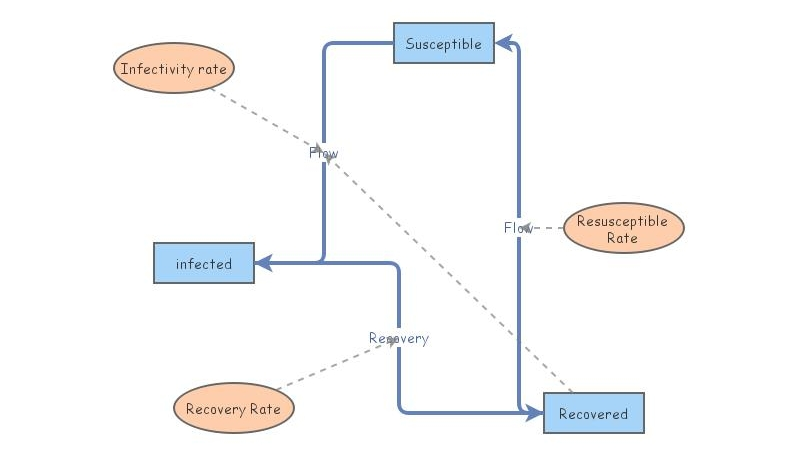A Simple SIR (Susceptible, Infected, Recovered) without infectionThis is a simple example of (part of a) simple SIR (Susceptible, Infected, Recovered) model, suggested by De Vries, et al. in A Course in Mathematical Biology.

They wanted to illustrate the comparative behavior of differential equations and discrete difference equations. We know that differential equations are generally solved numerically by discretizing them, so that the comparison is a little bit rigged....

A comparable model in Mathematica is available at
http://www.nku.edu/~longa/classes/2018spring/mat375/mathematica/SIRModel-w-discrete-version.nb